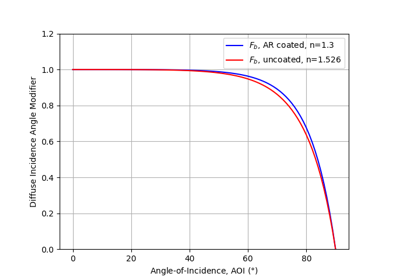# pvlib.iam.ashrae¶

pvlib.iam.ashrae(aoi, b=0.05)[source]

Determine the incidence angle modifier using the ASHRAE transmission model.

The ASHRAE (American Society of Heating, Refrigeration, and Air Conditioning Engineers) transmission model is developed in 1, and in 2. The model has been used in software such as PVSyst 3.

Parameters
• aoi (numeric) – The angle of incidence (AOI) between the module normal vector and the sun-beam vector in degrees. Angles of nan will result in nan.

• b (float, default 0.05) – A parameter to adjust the incidence angle modifier as a function of angle of incidence. Typical values are on the order of 0.05 .

Returns

iam (numeric) – The incident angle modifier (IAM). Returns zero for all abs(aoi) >= 90 and for all iam values that would be less than 0.

Notes

The incidence angle modifier is calculated as

$IAM = 1 - b (\sec(aoi) - 1)$

As AOI approaches 90 degrees, the model yields negative values for IAM; negative IAM values are set to zero in this implementation.

References

1

Souka A.F., Safwat H.H., “Determination of the optimum orientations for the double exposure flat-plate collector and its reflections”. Solar Energy vol .10, pp 170-174. 1966.

2

ASHRAE standard 93-77

3

PVsyst Contextual Help. https://files.pvsyst.com/help/index.html?iam_loss.htm retrieved on October 14, 2019

## Examples using pvlib.iam.ashrae¶